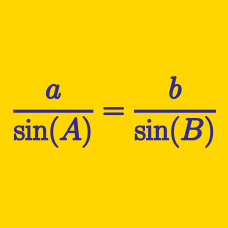Geometry

Solving Triangles - Problem Solving

In triangle $ABC$, $a=10$, $b=11$ and $c=12$. If $\cos A:\cos C$ can be expressed as an irreducible ratio $m:n$, where $m$ and $n$ are positive integers, what is the value of $m+n$?

Let $ABC$ be a triangle and let $a$, $b$ and $c$ be the lengths of the sides opposite to the vertices $A$, $B$ and $C$, respectively. If $a - 3b + c = 0$ and $2a + b - 2c = 0$, the ratio $\sin A : \sin B : \sin C$ can be expressed as $p:q:r$, where $p$, $q$ and $r$ are coprime positive integers. What is the value of $p+q+r$?

In a convex quadrilateral $ABCD,$ we are given the following three angles and side length: $\angle B=\angle D=90^\circ, \angle A=45^\circ, \lvert\overline{AC}\rvert= 3\sqrt{2}.$ What is the measure of the diagonal $\overline{BD} ?$

Alice and Bob start walking at the same time from the same spot towards their respective directions, forming a $60^\circ$ angle. Their speeds are $2$m and $3$m per second, respectively. If the distance between them $4$ seconds after the start can be expressed as $a\sqrt{b}$, where $b$ is prime number, what is $a+b$?

In triangle $ABC$, $\angle B=30^{\circ}$, $\angle C=90^{\circ}$, and $D$ is a point on side $BC$ such that $\overline{BD}=60$ and $\angle ADC=45^{\circ}$. The length of side $AC$ can be expressed as $p(1+\sqrt{3})$. What is the value of $p$?

×